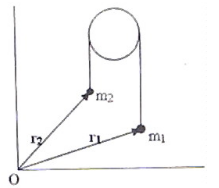Courses

# Test: Motion of Centre of Mass

## 10 Questions MCQ Test Physics For JEE | Test: Motion of Centre of Mass

Description
This mock test of Test: Motion of Centre of Mass for JEE helps you for every JEE entrance exam. This contains 10 Multiple Choice Questions for JEE Test: Motion of Centre of Mass (mcq) to study with solutions a complete question bank. The solved questions answers in this Test: Motion of Centre of Mass quiz give you a good mix of easy questions and tough questions. JEE students definitely take this Test: Motion of Centre of Mass exercise for a better result in the exam. You can find other Test: Motion of Centre of Mass extra questions, long questions & short questions for JEE on EduRev as well by searching above.
QUESTION: 1

### Two balls are thrown simultaneously in air. The acceleration of the centre of mass of the two balls, while in air

Solution:

The only force acting on the balls is force of gravity, so the acceleration of centre of mass is equal to g.

QUESTION: 2

### A shell following a parabolic path explodes somewhere in its flight. The centre of mass of fragments will continue to move in

Solution:

The internal forces have no effect on the trajectory of centre of mass, and the forces due to explosion are the internal forces. So the centre of mass will follow the same parabolic path even after explosion.

QUESTION: 3

### Two particles A and B of mass ‘m’ move towards each other under a mutual force of attraction. If velocity of A is 2 v and that of B is v, calculate the velocity of centre of mass of the system.

Solution:

The velocity of the centre of mass will be zero. The initial velocity of the particles were zero because both A and B were at rest so momentum will be zero. and it is given that there is no external force hence no change in momentum of the centre of mass and it will remain zero.
By the law of conservation of momentum, the momentum is zero, and mass is not zero, velocity must be zero

QUESTION: 4

Two bodies of masses m1 and m2 are moving such that their momentums are equal and opposite. The velocity of centre of mass of system of two bodies is:

Solution:

As momentum is p = mv and a vector quantity, if both are moving in opposite direction then the net momentum will be mv –mv. The minus sign shows that it is in opposite direction.
So p(net) is 0.

QUESTION: 5

Two spheres of masses m1 and m2 (m1>m2) respectively are tied to the ends of a light, inextensible string which passes over a light frictionless pulley. When the masses are released from their initial state of rest, the acceleration of their centre of mass is:Solution:

If, r1 and r2 are position vectors of the centres of the positional vector of their centre of mass is given by R=(m1r1+m2r2)/ (m1 + m2)
R= (m1r2+m2r2)/m1+m2)
The acceleration of the centre of mass is given by:
A=d2R/dt
= [m1 d2r/dt+m2 d2r/dt2 ]/(m1+m2)
But, d2r1/dt2 and d2r2/dt2 are the accelerations of masses m1 and m2
Have the same magnitude (m1-m2)/(m1+m2) g
If we take acceleration of [m1(m1-m2)/ (m1+m2)g – m2(m1-m2)/ (m1+m2)/]/ (m1+m2)
On simplifying that we get,
a=[(m1-m2)/ (m1+m2)]2 g

QUESTION: 6

When a fire cracker initially at rest, explodes into a number of fragments, the centre of mass

Solution:

When a fire cracker initially at rest, explodes into a number of fragments, the centre of mass remains stationary. This is analogous to the explosion of a ball while it is flying in a trajectory.

QUESTION: 7

A radioactive nucleus of mass M moving along the positive x-direction with speed v emits an alpha particle of mass m. If the alpha particle proceeds along the positive y-direction, the centre of mass of the system (made of the daughter nucleus and the alpha particle) will:

Solution:

The momentum of the initial particle remains conserved. Hence the emitted particles’ COM will remain along +ve x-axis.

QUESTION: 8

Which of the following options are correct,
where i, j and k are unit vectors along the x, y and z axis?

Solution:

Dot product of two different unit vectors is 0 and dot product of two same unit vectors is 1. Cross product of two different unit vectors taken according to right hand thumb rule is the other vector. Cross product of two same unit vectors is 0.

QUESTION: 9

There are two particles of masses m and 2m placed at a distance‘d’ apart on a smooth horizontal surface. Where will the collision occur (with respect to original positions), if they are allowed to move towards each other because of their mutual attraction?

Solution:

Their collision will occur at their center of mass.
If we consider body of mass 'm' at origin & '2m' at distance "d" from 1st body on X-axis then, center of mass,
X = m1(x1)+m2(x2)/m1+m2
X = m(0)+2m(d)/m+2m
X = 2md/3m
X = 2d/3
Hence A is the correct answer.

QUESTION: 10

A proton and an electron, initially at rest, are allowed to move under their mutal attractive force. Their centre of mass will:

Solution:

We require an external force to move the centre of mass of a system of particles. The mutual force of electrical attraction is an internal force which can not affect the position of the centre of mass of the system.